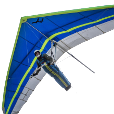# CIRCLE not drawn in the correct location

## Recommended PostsI have created a small DXF file to draw a line and a circle. The line is the unit normal vector (i.e. length 1 and perpendicular to the circle).

The line is drawn correctly but the circle is being drawn in the wrong location (the orientation is correct).

You can see below the 10, 20 & 30 codes for the line are the same as the 10, 20 & 30 codes for the circle.

When I report on the circle I get the following - center point, X=  -5.0552  Y=   1.3996  Z=   1.5996

I expect it to be center point, X=  -5.2394  Y=   1.5870  Z=   0.4437

I'm sure it's because I don't understand fully the DXF reference documentation - can anyone suggest what I'm doing wrong.

```0
SECTION
2
ENTITIES
0
LINE
8
L_VCL
10
-5.2304
20
1.5870
30
0.4437
11
-5.5690
21
0.6466
31
0.4738
0
CIRCLE
8
CL_BFC
10
-5.2304
20
1.5870
30
0.4437
40
0.2275
210
-0.3386
220
-0.9404
230
0.0301
0
ENDSEC
0
EOF```

##### Share on other sitesObject coordinate system is not equal World Coordinate System. Check 210, 220 and 230 DXF groups

Edited by kpblc

##### Share on other sitesThe following will convert the a point's WCS to an OCS if all you know is the Z axis of the Object's OCS.  Use the OCS coordinate for defining the circle's center in DXF.

```
(defun c:wcs2ocs (/)
; convert a WCS point to a OCS coordinate by defining an axis normal
; L. Minardi 11/8/2019
(command "ucs" "") ; set to WCS
(setq	p1    (getpoint "\npick start of normal vector")
p2    (getpoint p1 "\npick end of normal vector")
ptWCS (getpoint "\npick point to transform to OCS")
Az    (normalize (mapcar '- p2 p1))
)
; define OCS with Arbitrary Axis Alogorithm
(if (< (abs (nth 0 Az)) (/ 1.0 64.0))
(setq Ax (cross '(0 1 0) Az))
(setq Ax (cross '(0 0 1) Az))
)
(setq Ay (cross Az Ax))
(command "_.ucs"
"_non"
'(0 0 0)
"_non"
(trans Ax 0 1)
"_non"
(trans Ay 0 1)
)
(setq ptOCS (trans ptWCS 0 1))
(princ "\nWCS point: ")
(princ ptWCS)
(princ " = OCS point: ")
(princ ptOCS)
(princ)
)					; end defun
; normalize a vector
(defun normalize (v / d)
(setq	d (distance '(0 0 0) v)
v (mapcar '/ v (list d d d))
)
)

;;; cross product of 2 vectors a and b
(defun cross (a b / crs)
(setq	crs (list
(- (* (nth 1 a) (nth 2 b))
(* (nth 1 b) (nth 2 a))
)
(- (* (nth 0 b) (nth 2 a))
(* (nth 0 a) (nth 2 b))
)
(- (* (nth 0 a) (nth 1 b))
(* (nth 0 b) (nth 1 a))
)
)				;end list
)					;end setq crs
)					;end cross
```

##### Share on other sitesThe difference in the resulting position between the LINE & CIRCLE entities arises because the coordinates defining the LINE entity start & end points are defined relative to the World Coordinate System (WCS), and those defining the centre of a CIRCLE entity (and indeed, other planar entities) are defined relative to the Object Coordinate System (OCS) - such coordinate systems will only be equal for OCS planes parallel to the WCS plane.

You can transform coordinates from the WCS to the OCS using the Arbitrary Axis Algorithm, which is more conveniently implemented in the trans function:

```_\$ (trans '(-5.2304 1.5870 0.4437) 0 '(-0.3386 -0.9404 0.0301))
(-5.45875 0.435109 0.291967)```

Hence, your DXF data would become:

```0
SECTION
2
ENTITIES
0
LINE
8
L_VCL
10
-5.2304
20
1.5870
30
0.4437
11
-5.5690
21
0.6466
31
0.4738
0
CIRCLE
8
CL_BFC
10
-5.45875
20
0.435109
30
0.291967
40
0.2275
210
-0.3386
220
-0.9404
230
0.0301
0
ENDSEC
0
EOF```

##### Share on other sitesJust a dumb question why write the dxf do you have some program that you want to be able to make a Autocad dwg ?

Look at writing a script can be easier.

Is it for CNC but from say excel ?

Lastly there is open source to write a DWG.

Edited by BIGAL

##### Share on other sitesOn ‎11‎/‎9‎/‎2019 at 12:48 AM, BIGAL said:

why write the dxf do you have some program that you want to be able to make a Autocad dwg ?

Lastly there is open source to write a DWG.

I'm writing a program in VB.net to create a simple DXF file. I have been able to write lines, polylines, text, blocks etc. but am having trouble with Circle.

Is there open source available to do this?

In the past I have used Open Design Alliance to write a DWG file but their examples were for C++ and their support was either very good or erratic.

On ‎11‎/‎8‎/‎2019 at 7:24 PM, Lee Mac said:

You can transform coordinates from the WCS to the OCS using the Arbitrary Axis Algorithm, which is more conveniently implemented in the trans function:

I could try to recreate the trans function in VB.net - it looks difficult.

## Join the conversation

You can post now and register later. If you have an account, sign in now to post with your account.
Note: Your post will require moderator approval before it will be visible.×   Pasted as rich text.   Paste as plain text instead

Only 75 emoji are allowed.